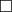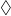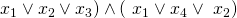# Monthly Archives: January 2020

## Modal Logic S5-Satisfiability

One of the things that strikes me about the logic chapter is that the problems all seem to be either “trivial” or “really, really, hard”.  I just hope I’m classifying them correctly.  Here’s one that I think is a trivial one.

The problem: Modal Logic S5-Satisfiability.  This is problem LO13 in the appendix.

The description:  Given a set of variables, and a modal logic formula over those variables, is it “S5-Satisfiable”?  I think that “S5-Satisfiable” is just the regular notion of satisfiability extended to the modal operators.

Example/Reduction: I think all we need to know about Modal Logic for our purposes can be found in from this Wikipedia article. In it, they say that Modal Logic extends propositional or predicate logic by adding two new operations:

•which means “Necessarily”.  SoP means “it is necessary that P is true”.
•which means “Possibly”.  SoP means “it is possible that P is true”.

Since you can definein terms of, G&J just have the diamond symbol in the language.

People use Modal logic to think about “worlds” in which facts are necessarily or possibly true, and different agents know different things.  I talk about it (vaguely) when I teach Artificial Intelligence because it can model things agents know, and things agents know that other agents know, and so on.  (The Russell and Norvig AI book has a fun example about how Superman knows that Clark Kent is Superman, but Lois Lane doesn’t know.  And Superman knows that Lois doesn’t know.  But he knows that Lois knows that someone is Superman).

Notice, though, that for our purposes, we don’t need the modal operators.  So, for example, a regular 3SAT formula: (is a modal logic formula (and, in this case, is true by setting all 4 variables to true).  So the reduction from 3SAT is just “Take a 3Sat formula, keep it the same, call it a modal logic formula.  Obviously, this is satisfiable iff the original (unchanged) formula is”.

Difficulty: 2.  Maybe it should be 1, but you need to at least think a little about what you’re leaving out.

## First Order Theory of Equality

LO11 is QBF

This next problem is related to QBF and is proved in a similar way.

The problem: First Order Theory of Equality.  This is problem LO12 in the appendix.

The description: Given a set U of variables {u1..un}, and a sentence S over U in the first order theory of equality.  Is S true in all models of the theory?

The first order theory of equality defines logical operations for equality (on variables), and the standard ∧, ∨, ¬, → operations (on expressions).  It also lets us define ∀ and ∃ quantifiers on variables outside of an expression.

Example: My lack of mathematical logic knowledge is again letting me down as I’m not entirely sure what the difference between “models of the theory” and “assignments of truth values” is.  It seems to me that this is very similar to QBF, with the addition of equality. So I think we can ask whether an expression like:

∀x ∀y ∀z (x=y) → (x = z) is always true.  (It is).

I think, at least, this is the meaning that the Stockmeyer and Meyer paper use.

Reduction: Stockmeyer and Meyer again do a “generic transformation” using Turing Machines.  What they do is the generic transformation for QBF (it’s Theorem 4.3 in the paper), and then show this problem is a corollary to it because it follows from the “well-known fact that a first-order formula with n quantifiers and no predicates other than equality is valid iff it is valid for domains of all cardinalities between 1 and n”.

It’s not a well-known fact to me, so I guess I’ll just trust them.

Difficulty: I guess it depends on just how “well-known” that fact is for your class.  If I gave the QBF reduction a 3, maybe this is a 5?  But if you have to tell them the fact, maybe it’s too obvious?  I don’t know.

## Truth-Functionally Complete Connectives

This is another “private communication” problem that I couldn’t find an obvious reduction for online.  I’ve used the holidays as an excuse to not put up a post so I could work on this problem, but even with the extra time, this problem has stumped me.

The problem: Truth-Functionally Complete Connectives.  This is problem LO10 in the appendix.

The description: Given a set U of variables, and a set C of well-formed Boolean expressions over U, is C truth-functionally complete?  In other words, can we find a finite set of unary and binary operators D = {θ1..θk} such that for each θi we can find an expression E in C, and a substitution s: U->{a,b} such that s(E) ≡ aθib (if θi is binary) or s(E) ≡ θia (if θi is unary)?

Example: So, “Truth-Functionally Complete” means that you can use the operations in D to generate all possible truth tables.  So some possible candidates for D are {AND, NOT} or just {NAND}.

So, if U = {x,y} and C = {~x, x∧y}, then I can just choose D to be {NOT, AND} and use the obvious substitution.  I think the hard part here is that the problem asks if such a D exists- I think the substitutions are probably always straightforward, once you get the functionally complete set D that gives you the best mapping..

Reduction: As I said above, this is a “Private Communication” problem, and so after a lot of searching, I found this paper by Statman, which I’m honestly am not even sure is the correct one. It at least talks about substitutions and deterministic polynomial time algorithms and things, but it very quickly goes into advanced Mathematical Logic that I wasn’t able to follow.  If someone out there knows more about this than I do and wants to chime in, let me know!

Edited Jan 22, 2020: Thanks to Thinh Nguyen for the help in coming up with the idea for the reduction- please check it out in the comments!

Difficulty: 10.  This is why we have the level 10 difficulty- for problems I can’t even follow.